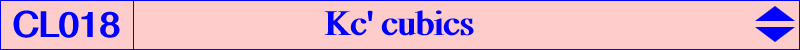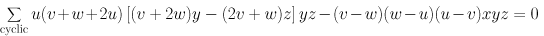Let W = (p : q : r) be a point. Kp(W) is the locus of pivots of all pK+ invariant under the isoconjugation with pole W. These cubics form the class CL014 of cubics. The locus of the common point Q of the three asymptotes is Kc(W), member of the class CL015. Now, instead of fixing the pole W, we fix the pivot P = (u : v : w). The locus of poles of all pK+ with pivot P is Kw(P) and the locus of the common point Q of the three asymptotes is Kc'(P). The cubics Kw(P) and Kc'(P) form the classes CL017 and CL018 respectively. Kc'(P) is always a K+ i.e. its asymptotes are always concurrent at the point with coordinates : (v + w)(v + w + 2u)[5u (u + v + w) + 3vw] : : . The general equation of Kc'(P) is :Kc'(P) contains the points Qi intersections of the asymptotes of the cubic Kw(Wi) of the class CL017.

Kc'(P) contains :

• A, B, C.

• Qa = 0 : 2v + w : v + 2w, homothetic of the cevian Pa of P under h(Ma,1/3) where Ma is the midpoint of BC. Qb, Qc similarly.

• G (centroid of ABC) = Q0.

• Q1 = v + w + 2u : : , homothetic of P under h(G,1/4) and complement of complement of P.

• Q2

• Q3 = P (this is the case of the degenerate cubic of CL017).

• Q4 = u(v + w)(v + w + 2u) : : , the centroid of the cevian triangle of P.

• Q5 = (v^2 + w^2 - u^2 + vw) / (v^2 + w^2 - u^2 + vw + uv + uw) : : .

• Q6 = (v + w + 2u)(v^2 + w^2 - u^2 + vw) / (v^2 + w^2 - u^2) : : .

• Q7 = u / (u^2 + vw + 2uv + 2uw) : : .

• Q8 =

• Q9 = (v + w + 2u) / [u(v + w - u) + 2vw] : : .

• Q10 = u(v + w - u) / (v + w) : : .

• Q11 = u(v + w + 2u)(v^2 + w^2 + vw -uv - uw) / (v + w - u) : : .

• Q12

• Q13

 alignements Q0 Q1 Q3 Q0 Q4 Q7 Q0 Q6 Q10 Q1 Q2 Q7 Q1 Q5 Q10 Q1 Q6 Q11 Q1 Q8 Q9 Q2 Q9 Q10 Q3 Q4 Q10 Q3 Q7 Q8 Q3 Q9 Q13 Q4 Q9 Q11 Q5 Q7 Q11 Q6 Q7 Q13 Q7 Q10 Q12Special cases 1. This class contains only one equilateral cubic : Kc'(X4) = K412. 2. Kc'(G) decomposes into the medians of ABC. 3. Kc'(P) is a nK if and only if either : – P lies at infinity but the cubic degenerates. – P lies on the Steiner ellipse. The pole of the nK lies on the homothetic of the Steiner ellipse under h(G, 1/4) and on the cubic. The root is the infinite point of the conjugated diameter of the line tP-G in the Steiner ellipse. For axample, Kc'(X99) = nK(X620, X524, X2). 4. For any point P on K080 = KO++, there is always an isogonal pK with the same asymptotic directions as Kc'(P).